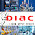### Oracle Financial Services-Aptitude Questions - Recruitment-2012 - ShredskeralaOracle Financial Services -
All Kerala Mega Campus Recruitment - 2012
The Aptitude test for Oracle Financial Services - All Kerala Mega Campus Recruitment - 2012 batch on 4th November  at Sree Narayana Guru College of Engineering & Technology, Payyanur, Kannur, was an on-line multiple choice test and was focused on  coding skills, basic computer knowledge & engineering aptitude skills. It had 3-major sections -   Software engineering, Basic computer knowledge & English written.

Software Engineering Aptitude Section – Sample Questions (1/4)

The Software Engineering Aptitude Section is composed of 35 questions divided into 4 sections used to evaluate your fundamental knowledge and skills in Mathematical Reasoning, IT Reasoning, Attention to Detail and Logic Diagramming.

You will have 35 minutes to complete this assessment. Please note that once you have completed a question you will NOT be able to return to it in order to make changes. No points will be deducted for wrong answers.

Assessment Breakdown:
 Section Time Questions Math Reasoning 10 Minutes 10 IT Reasoning 10 Minutes 10 Attention to Detail 5 Minutes 10 Logic Diagramming 10 Minutes 5 Total 35 Minutes 35 Questions

Math Reasoning section:

It is designed to test your ability to understand and implement mathematical principles within concrete circumstances.You will have 10 minutes to complete the 10 questions in this section.

Question Number 1

If n is divided by 5, the remainder is 4. If 5 divided by 2n the remainder will be __________.

a. 1

b. 3

c. 4

d. 2

Question Number 2

A train 150 meters long crosses a pole in 7.5 seconds. Another train of the same length crosses a platform, 250 meters long, in 40 seconds. What is the difference in the distances covered by the two trains in 2 hours?

a. 72 km

b. 36 km

c. 54 km

d. 18 km

Question Number 3

A pipe 98 inches long is cut such that the shorter piece is 5/9ths of the longer piece. If the longer piece is further cut in the same proportion, what would be the length of the longer piece resulting from the second cut?

a. 22.5 inches

b. 30.5 inches

c. 36.5 inches

d. 40.5 inches

Question Number 4

Multiplying a number by 2 is the same as dividing by __________.

a. 1/4

b. 4

c. .5

d. .2

Question Number 5

Amy spends 70% of her income on household expenditure, 60% of the remaining on the education of her children and then 40% of the remaining is given to her old mother. Finally, she has US \$576 in her hand. Her salary is __________.

a. US \$6000

b. US \$8000

c. US \$9000

d. US \$10000

Question Number 6

A bag contains 5 red balls and 4 white balls. If two balls are drawn from the bag, then the probability that both the balls are of the same color is__________.

a. 3/18

b. 13/18

c. 4/9

d. 5/9

Question Number 7

Two trains start at the same time from New York and Chicago and proceed towards each other at 42 km/hr & 48 km/hr respectively. When they meet, it is found that one train had traveled 42 km more than the other train. The distance between the two stations is __________.

a. 630 km

b. 750 km

c. 720 km

d. 540 km

Question Number 8

A can do a piece of work in 42 days and B can do the work in 35 days. B works for two days, then A joins him. In how many days in all, is the work completed?

a. 22

b. 18

c. 16

d. 20

Question Number 9

The present population of a village is 16,000. If it increases at the rate of 5% per year, then what will be the population two years hence?

a. 18,025

b. 16,975

c. 17,640

d. 16,960

Question Number 10

In a ratio equal to 4 : 9, the antecedent is 36, the consequent is __________.

a. 81

b. 76

c. 16

d. 64

1.Thank you so much... i didn’t have the knowledge in this now i get an idea about this.. thks a lot:-) Also do you want cash for credit card in chennai at low interest.

2.DIAC is an ISO 9001: 2008 Industrial Automation Training Institute and providing 100% Placement in core companies for the engineering graduates.